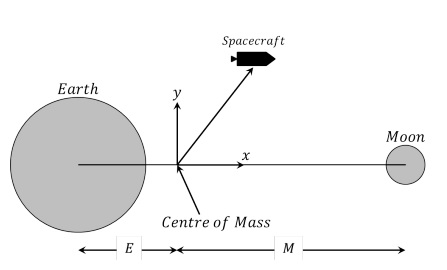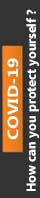We're Open

0% Plagiarism Guaranteed & Custom Written

# Your job as modellers is to write a Matlab program which prompts the user for the length of time you wish to solve the spacecraft motion for, the initial position and the initial velocity of the spacecraft

This assignment is designed to test your ability to use Matlab to solve a mathematical modelling problem. You are asked to write a Matlab program, which can include scripts and function les which you write. The program should be user friendly i.e., your program should require very little knowledge of programming or mathematical modelling from the user in order to use/run it. You should make comments within your program so it is clear to anyone using it what each part of your program does. This assignment counts for 30% of your nal grade for this module.

The marks will be distributed as follows:

• 10 marks will be awarded for writing a program which successfully solves the given problem and displays the solution graphically.

• 10 marks will be awarded for the presentation of your program. Is it easy to read? Are there comments correctly describing the various components in both the script and any functions made. Is the program ecient? Does using the help function on your program adequately describe what it does and what is required from the user.

• 10 marks will be awarded for how user friendly your program is and your understanding of it. Each student will come to the front of the class and demonstrate their program (See Canvas for presentation date). This will take less than 5 minutes and is for me to judge how well you understand your program and that it is your own work.

Failure to demonstrate your program, on the date specied, will be interpreted as failure to submit and you will be awarded zero marks for the assignment. On the next pages the modelling problem is set up for you so you don`t need to understand how to derive this model, you just need to apply the techniques you have learned in the Matlab sessions to solve it in Matlab.

The Three Body Problem

Let us consider the motion of a spacecraft in the gravitational eld of two bodies (such as the Earth and the Moon). The equations governing the motion of the spacecraft form a system of second order dierential equations. The system is illustrated below.Figure 1: The Three Body Problem. E and M are the distances the Earth and Moon are from the centre of mass respectively. Both bodies impose a force on the spacecraft according to Newton`s gravitational law, but the mass of the spacecraft is too small to signicantly aect the motion of the bodies. We therefore neglect the inuence of the spacecraft on the two stellar bodies. Our coordinate system has its origin at the centre of mass of the Earth and Moon. The governing equations are given by d 2x dt2 = 2 dy dt + x − M(x + E) r 3 E − E(x − M) r 3 M d 2 y dt2 = −2 dx dt + y − My r 3 E − Ey r 3 M where rE = p (x + E) 2 + y 2 , rM = p (x − M) 2 + y 2 , and M = 1 − E. The Earth and Moon are assumed to have circular orbits around the centre of mass of the system. To simplify the problem, we therefore consider a coordinate system which is rotating with the Earth and Moon. In this system, the Earth does not move and is located at (−E, 0) and the moon is located at (M, 0). The governing equations contain terms in 1 r 2 , which correspond to the gravitational force. (Ok, in the governing equations it is actually 1 r 3 , but this term is still associated with the gravitational force, ask me if you would like an explanation.) The equations also contain terms dy dt and − dx dt , which correspond to the Coriolis force, as well as terms x and y, which correspond to the Centrifugal force. Before you try and solve this system of second-order dierential equations we must reduce it to a set of four rst order equations. To do this, we dene z1 = x, z2 = dx dt , z3 = y, and z4 = dy dt . 2 In vector form, we get Z =      z1 z2 z3 z4      =      x x˙ y y˙      . Therefore, Z˙ =      z˙1 z˙2 z˙3 z˙4      =      x˙ x¨ y˙ y¨      =       z2 2z4 + z1 − M(z1+E) r 3 E − E(z1−M) r 3 M z4 −2z2 + z3 − Mz3 r 3 E − Ez3 r 3 M       . where rE = p (z1 + E) 2 + z 2 3 , rM = p (z1 − M) 2 + z 2 3 , and M = 1 − E. Set the value E = 0.012277. Your Assignment Your job as modellers is to write a Matlab program which prompts the user for the length of time you wish to solve the spacecraft motion for, the initial position and the initial velocity of the spacecraft. Use the following values to test your program: Time from: t = 0 to t = 24. Initial Position: x(0) = 1.15, y(0) = 0. Initial Velocity: dx dt (0) = 0, dy dt(0) = 0.008688. After establishing these values your program will solve the given system of equations and plot the solution (the trajectory of the spacecraft) in a gure window. The solution for the test parameters above is plotted in the gure below. You should be able to reproduce this plot with you program. Figure 2: Solution to the Three Body Problem for the test parameters.

100% Plagiarism Free & Custom Written,International House, 12 Constance Street, London, United Kingdom,
E16 2DQ

## STILL NOT CONVINCED?

We've produced some samples of what you can expect from our Academic Writing Service - these are created by our writers to show you the kind of high-quality work you'll receive. Take a look for yourself!FLAT 25% OFF ON EVERY ORDER.Use "FLAT25" as your promo code during checkout